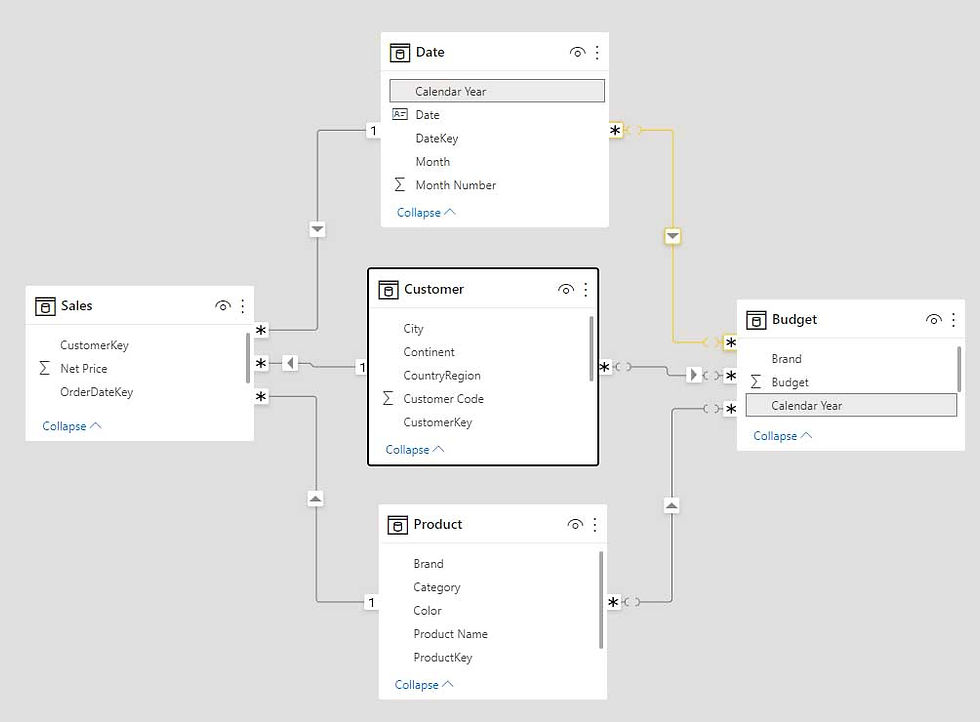top of page
Search
• Marek Vavrovic

# Compound Annual Growth Rate (CAGR) using DAX

Updated: May 2, 2022

## What Is the Compound Annual Growth Rate (CAGR)?

The compound annual growth rate (CAGR) is the rate of return (RoR) that would be required for an investment to grow from its beginning balance to its ending balance, assuming the profits were reinvested at the end of each period of the investment’s life span.

## What the CAGR Can Tell You?

The compound annual growth rate isn’t a true return rate, but rather a representational figure. It is essentially a number that describes the rate at which an investment would have grown if it had grown at the same rate every year and the profits were reinvested at the end of each year.

Data

Working with 2 tablesOverview

Step 1:

Calculate CAGR using formula:

(End Value/Start Value)^(1/Periods) -1

In the example above it comes up to 11.8%

Step 2:

Calculate Forecast.

Revenue_2018 = Revenue_2017 *(1+CAGR)^1

Revenue_2019 = Revenue_2017 *(1+CAGR)^2

Revenue_2020 = Revenue_2017 *(1+CAGR)^3

Revenue_2021 = Revenue_2017 *(1+CAGR)^4

Revenue_2022 = Revenue_2017 *(1+CAGR)^5

Revenue_2023 = Revenue_2017 *(1+CAGR)^6

## Solution

1. calculate the value of for the first year

FirstYear =

FIRSTNONBLANK (

ALL ( 'cagr year'[Year] ),

CALCULATE ( SUM ( 'cagr revenue'[revenue]) )

)

2. calculate the value of last year

LastYear =

LASTNONBLANK (

ALL ('cagr year'[Year]),

CALCULATE ( SUM ( 'cagr revenue'[revenue] ) )

)3. Calculate CAGR: (End Value/Start Value)^(1/Periods) -1

CAGR =

VAR Lastyear = [LastYear]

VAR Firstyear = [FirstYear]

VAR No_of_Years = [LastYear] - [FirstYear]

RETURN

POWER (

DIVIDE (

CALCULATE ( SUM ( 'cagr revenue'[revenue] ), 'cagr year'[Year] = Lastyear ),

CALCULATE ( SUM ( 'cagr revenue'[revenue] ), 'cagr year'[Year]= Firstyear )

),

1 / No_of_Years

)

- 1

4. Calculate forecast

Forecast =

VAR Lastyear = [LastYear]

VAR No_of_Years =

SELECTEDVALUE ( 'cagr year'[Year] ) - [LastYear]

RETURN

IF (

SELECTEDVALUE ( 'cagr year'[Year] ) > Lastyear,

CALCULATE ( SUM ( 'cagr revenue'[revenue] ), 'cagr year'[Year] = Lastyear )

* POWER ( ( 1 + [CAGR] ), No_of_Years )

)5. Build a stunning visualisation acceptable by your client.## Power BI Budgeting

Sample data:

I have a Budget table with 3 scenarios (Low, Medium, High) and 3 countriesCreating a relationship to Budget table.

Customer - Budget: many-to-many

Product - Budget: many-to-many

Date - Budget: many-to-manyCardinality: many to many

Cross filter direction: SingleThis calculated column in Badget table was used to create a relationship between Date and Budget table.I am not going to make the relationship this way. I create a new Budget table that will have its values distributed based on the sales ratio in 2009. This table will be summarized by Brand, Country Region, and Month Number, and the values will be distributed by the percentage sales in 2009 for each Country, Region, and Month Number.

Assign Budget =

CALCULATETABLE(

CROSSJOIN(

SUMMARIZE(

Sales,

'Product'[Brand],

Customer[CountryRegion],

'Date'[Month Number]),

ALLNOBLANKROW(Budget[Scenario])),

"Date", DATE(2010, 'Date'[Month Number],1), "Assign Budget",

DIVIDE ([Sales],

CALCULATE([Sales],REMOVEFILTERS('Date'[Month Number])))

*

LOOKUPVALUE(Budget[Budget],

Budget[Brand],Product[Brand],

Budget[CountryRegion], Customer[CountryRegion],

Budget[Scenario],Budget[Scenario])),

FILTER(ALLNOBLANKROW('Date'[Calendar Year]),

'Date'[Calendar Year]="CY 2009")

Now I have one-to-may relationship between Date and Budget table.Next relationship I need to improve is the relationship between Customer and Budget tables.

CustomerRegion table is now able to filter Customer and Badget tbls.

CountryRegion = DISTINCT(

UNION(

ALLNOBLANKROW(Customer[CountryRegion]),

ALLNOBLANKROW(Budget[CountryRegion]) )

)For Product - AssignBudget relationship I could you the limited relationship:

Cardinality: many to many

Cross filter direction: Single

or create a bridge table with unique values....this is not finished yet...